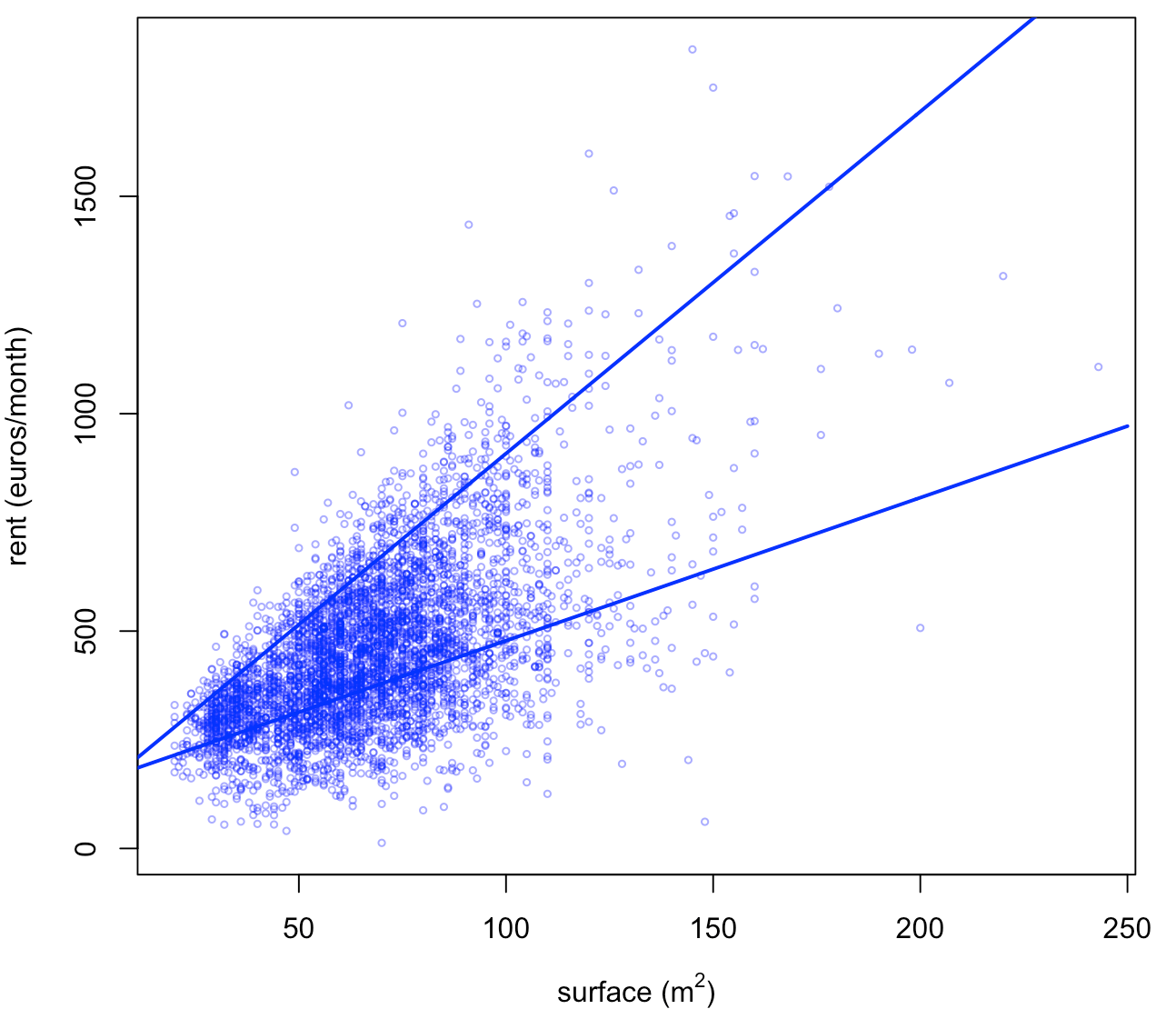Want to share your content on R-bloggers? click here if you have a blog, or here if you don't.

After my series of post on classification algorithms, it’s time to get back to R codes, this time for quantile regression. Yes, I still want to get a better understanding of optimization routines, in R. Before looking at the quantile regression, let us compute the median, or the quantile, from a sample.

## Median

Consider a sample $\{y_1,\cdots,y_n\}$. To compute the median, solve$$\min_\mu \left\lbrace\sum_{i=1}^n|y_i-\mu|\right\rbrace$$which can be solved using linear programming techniques. More precisely, this problem is equivalent to$$\min_{\mu,\mathbf{a},\mathbf{b}}\left\lbrace\sum_{i=1}^na_i+b_i\right\rbrace$$with $a_i,b_i\geq 0$ and $y_i-\mu=a_i-b_i$, $\forall i=1,\cdots,n$.
To illustrate, consider a sample from a lognormal distribution,

n = 101
set.seed(1)
y = rlnorm(n)
median(y)
 1.077415

For the optimization problem, use the matrix form, with $3n$ constraints, and $2n+1$ parameters,

library(lpSolve)
A1 = cbind(diag(2*n),0)
A2 = cbind(diag(n), -diag(n), 1)
r = lp("min", c(rep(1,2*n),0),
rbind(A1, A2),c(rep(">=", 2*n), rep("=", n)), c(rep(0,2*n), y))
tail(r$solution,1)  1.077415 It looks like it’s working well… ## Quantile Of course, we can adapt our previous code for quantiles tau = .3 quantile(x,tau) 30% 0.6741586 The linear program is now$$\min_{\mu,\mathbf{a},\mathbf{b}}\left\lbrace\sum_{i=1}^n\tau a_i+(1-\tau)b_i\right\rbrace$$with $a_i,b_i\geq 0$ and $y_i-\mu=a_i-b_i$, $\forall i=1,\cdots,n$. The R code is now A1 = cbind(diag(2*n),0) A2 = cbind(diag(n), -diag(n), 1) r = lp("min", c(rep(tau,n),rep(1-tau,n),0), rbind(A1, A2),c(rep(">=", 2*n), rep("=", n)), c(rep(0,2*n), y)) tail(r$solution,1)
 0.6741586

So far so good…

## Quantile Regression (simple)

Consider the following dataset, with rents of flat, in a major German city, as function of the surface, the year of construction, etc.

base=read.table("http://freakonometrics.free.fr/rent98_00.txt",header=TRUE)

The linear program for the quantile regression is now$$\min_{\mu,\mathbf{a},\mathbf{b}}\left\lbrace\sum_{i=1}^n\tau a_i+(1-\tau)b_i\right\rbrace$$with $a_i,b_i\geq 0$ and $$y_i-[\beta_0^\tau+\beta_1^\tau x_i]=a_i-b_i$$$\forall i=1,\cdots,n$. So use here

require(lpSolve)
tau = .3
n=nrow(base)
X = cbind( 1, base$area) y = base$rent_euro
A1 = cbind(diag(2*n), 0,0)
A2 = cbind(diag(n), -diag(n), X)
r = lp("min",
c(rep(tau,n), rep(1-tau,n),0,0), rbind(A1, A2),
c(rep(">=", 2*n), rep("=", n)), c(rep(0,2*n), y))
tail(r$solution,2)  148.946864 3.289674 Of course, we can use R function to fit that model library(quantreg) rq(rent_euro~area, tau=tau, data=base) Coefficients: (Intercept) area 148.946864 3.289674 Here again, it seems to work quite well. We can use a different probability level, of course, and get a plot plot(base$area,base$rent_euro,xlab=expression(paste("surface (",m^2,")")), ylab="rent (euros/month)",col=rgb(0,0,1,.4),cex=.5) sf=0:250 yr=r$solution[2*n+1]+r$solution[2*n+2]*sf lines(sf,yr,lwd=2,col="blue") tau = .9 r = lp("min", c(rep(tau,n), rep(1-tau,n),0,0), rbind(A1, A2), c(rep(">=", 2*n), rep("=", n)), c(rep(0,2*n), y)) tail(r$solution,2)
 121.815505   7.865536
yr=r$solution[2*n+1]+r$solution[2*n+2]*sf
lines(sf,yr,lwd=2,col="blue")## Quantile Regression (multiple)

Now that we understand how to run the optimization program with one covariate, why not try with two ? For instance, let us see if we can explain the rent of a flat as a (linear) function of the surface and the age of the building.

require(lpSolve)
tau = .3
n=nrow(base)
X = cbind( 1, base$area, base$yearc )
y = base$rent_euro A1 = cbind(diag(2*n), 0,0,0) A2 = cbind(diag(n), -diag(n), X) r = lp("min", c(rep(tau,n), rep(1-tau,n),0,0,0), rbind(A1, A2), c(rep(">=", 2*n), rep("=", n)), c(rep(0,2*n), y)) tail(r$solution,3)
 0.000000 3.257562 0.077501

Unfortunately, this time, it is not working well…

library(quantreg)
rq(rent_euro~area+yearc, tau=tau, data=base)
Coefficients:
(Intercept)         area        yearc
-5542.503252     3.978135     2.887234

Results are quite different. And actually, another technique can confirm the later (IRLS – Iteratively Reweighted Least Squares)

eps = residuals(lm(rent_euro~area+yearc, data=base))
for(s in 1:500){
reg = lm(rent_euro~area+yearc, data=base, weights=(tau*(eps>0)+(1-tau)*(eps<0))/abs(eps))
eps = residuals(reg)
}
reg$coefficients (Intercept) area yearc -5484.443043 3.955134 2.857943  I could not figure out what went wrong with the linear program. Not only coefficients are very different, but also predictions… yr = r$solution[2*n+1]+r$solution[2*n+2]*base$area+r$solution[2*n+3]*base$yearc
plot(predict(reg),yr)
abline(a=0,b=1,lty=2,col="red")It’s now time to investigate….